### BaseMap 画世界地图

BaseMap 画世界地图，主要方法是画地球海岸线，画国家分界线主要方法是：`m.drawcoastlines()`

BaseMap 画地球地图详细代码如下：

 `1234567891011` ``````import matplotlib.pyplot as plt from mpl_toolkits.basemap import Basemap import numpy as np def drawcoast(): plt.figure(figsize=(12, 8)) m = Basemap() # 创建一个地图 m.drawcoastlines() # 画海岸线 plt.show() # 显示图像 if __name__ == '__main__': drawcoast() ``````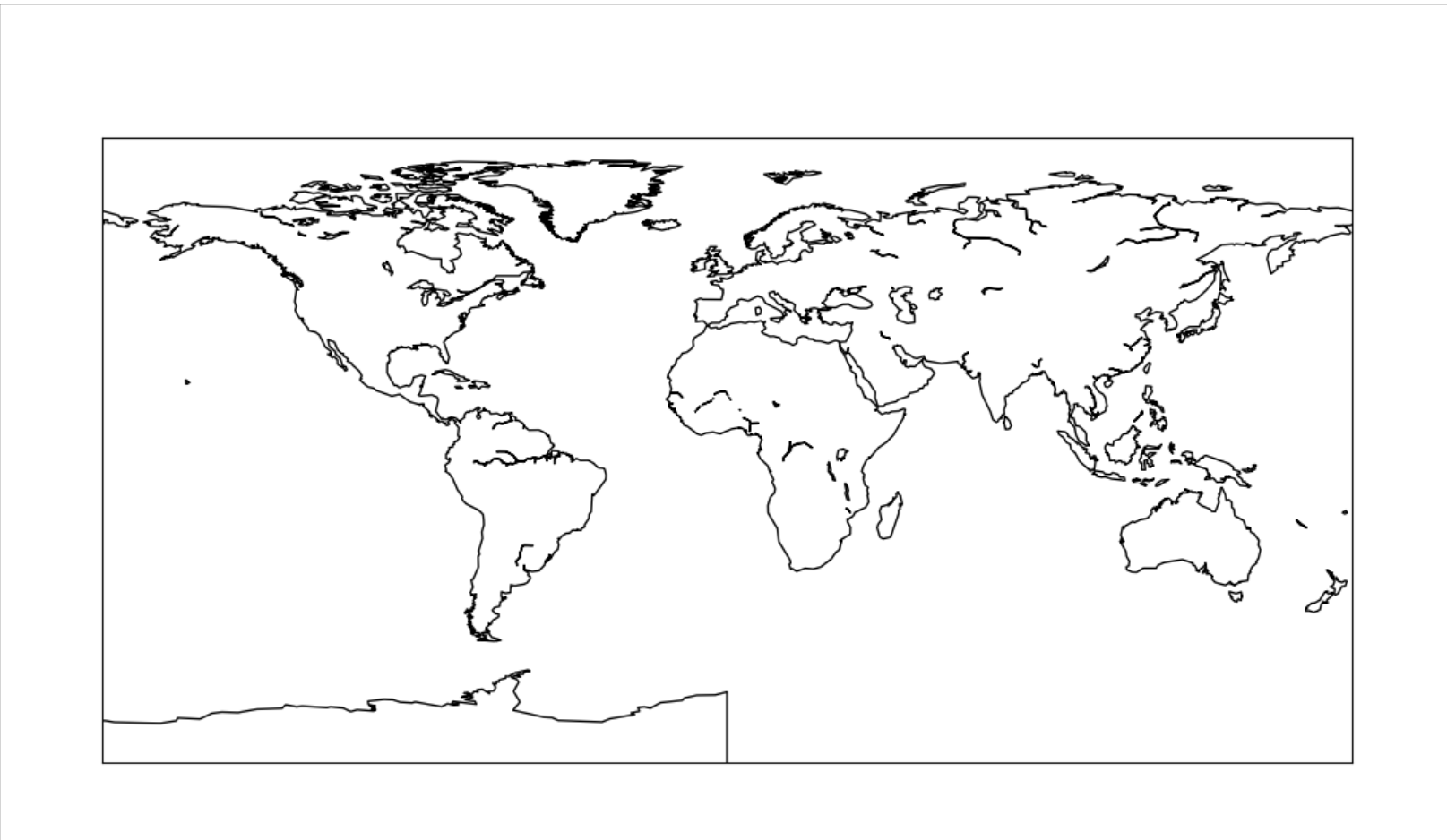### 用 BaseMap 画中国地图

 `12345678` ``````# 画中国地图 def draw_china(): plt.figure(figsize=(10, 6)) m = Basemap(llcrnrlon=77, llcrnrlat=14, urcrnrlon=140, urcrnrlat=51, projection='lcc', lat_1=33, lat_2=45, lon_0=100) m.drawcountries(linewidth=1.5) m.drawcoastlines() plt.show() ``````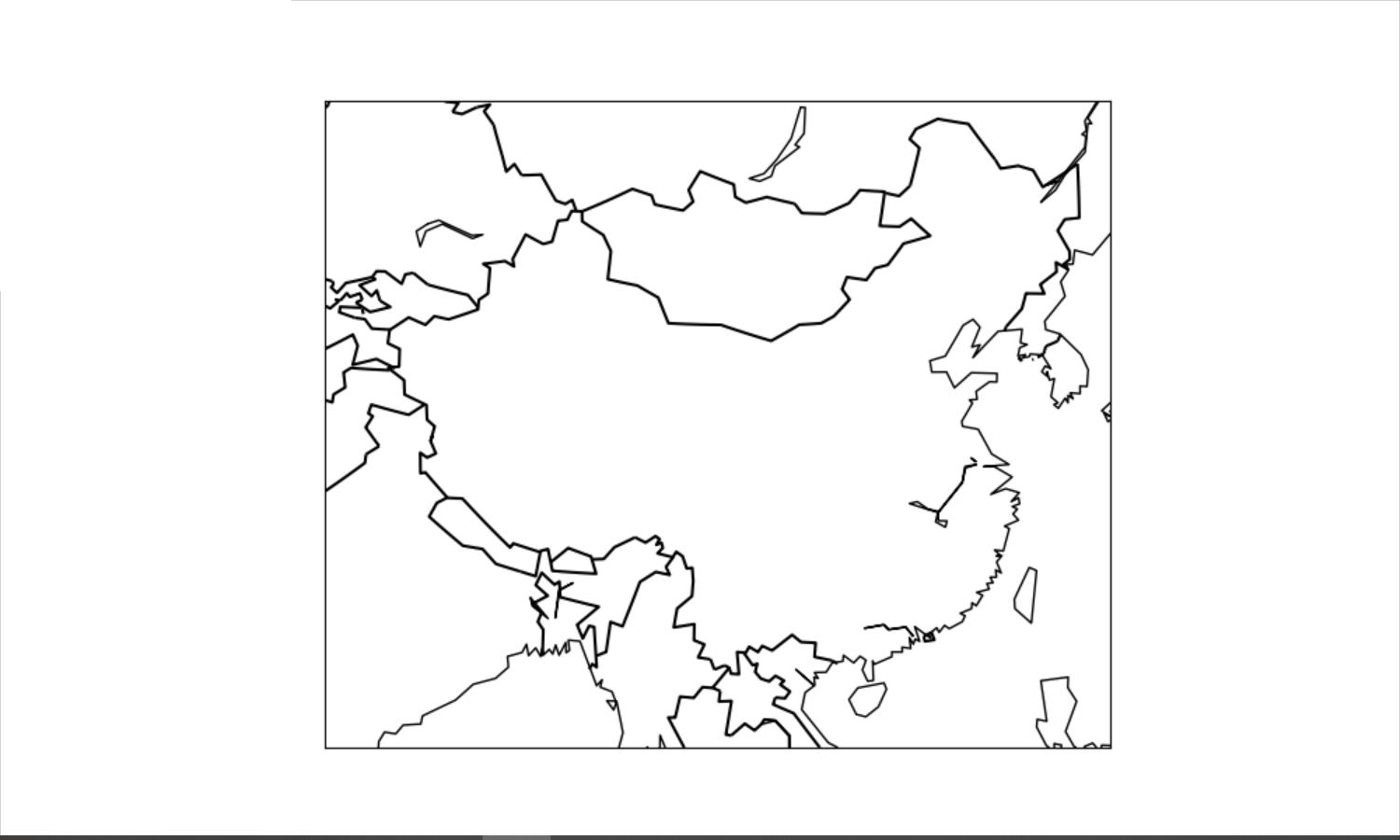### 用 BaseMap 绘制地球

 `123456` ``````def draw_basic(): map = Basemap(projection='ortho', lat_0=0, lon_0=0) map.drawmapboundary(fill_color='aqua') map.fillcontinents(color='gray',lake_color='aqua') map.drawcoastlines() plt.show() ``````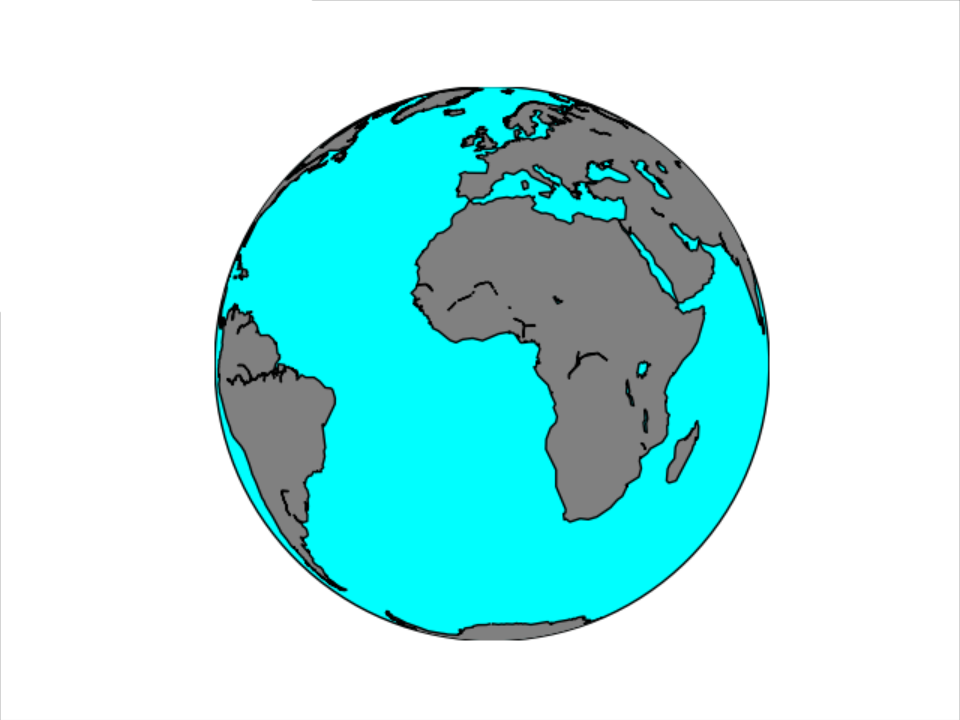### 用 BaseMap 绘制人口分布图

 `1234567891011121314151617181920212223242526272829303132333435363738394041424344454647484950` ``````def drawearth(): names = [] pops = [] lats = [] lons = [] countries = [] file = open("data/main_city", encoding='utf-8').readlines() for line in file: info = line.split() names.append(info) pops.append(float(info)) lat = float(info[:-1]) if info[-1] == 'S': lat = -lat lats.append(lat) lon = float(info[:-1]) if info[-1] == 'W': lon = -lon + 360.0 lons.append(lon) country = info countries.append(country) # set up map projection with # use low resolution coastlines. map = Basemap(projection='ortho', lat_0=35, lon_0=120, resolution='l') # draw coastlines, country boundaries, fill continents. map.drawcoastlines(linewidth=0.25) map.drawcountries(linewidth=0.25) # draw the edge of the map projection region (the projection limb) map.drawmapboundary(fill_color='#689CD2') # draw lat/lon grid lines every 30 degrees. map.drawmeridians(np.arange(0, 360, 30)) map.drawparallels(np.arange(-90, 90, 30)) # Fill continent wit a different color map.fillcontinents(color='#BF9E30', lake_color='#689CD2', zorder=0) # compute native map projection coordinates of lat/lon grid. x, y = map(lons, lats) max_pop = max(pops) # Plot each city in a loop. # Set some parameters size_factor = 80.0 y_offset = 15.0 rotation = 30 for i, j, k, name in zip(x, y, pops, names): size = size_factor * k / max_pop cs = map.scatter(i, j, s=size, marker='o', color='#FF5600') plt.text(i, j + y_offset, name, rotation=rotation, fontsize=10) plt.title('earth') plt.show() if __name__ == '__main__': drawearth() ``````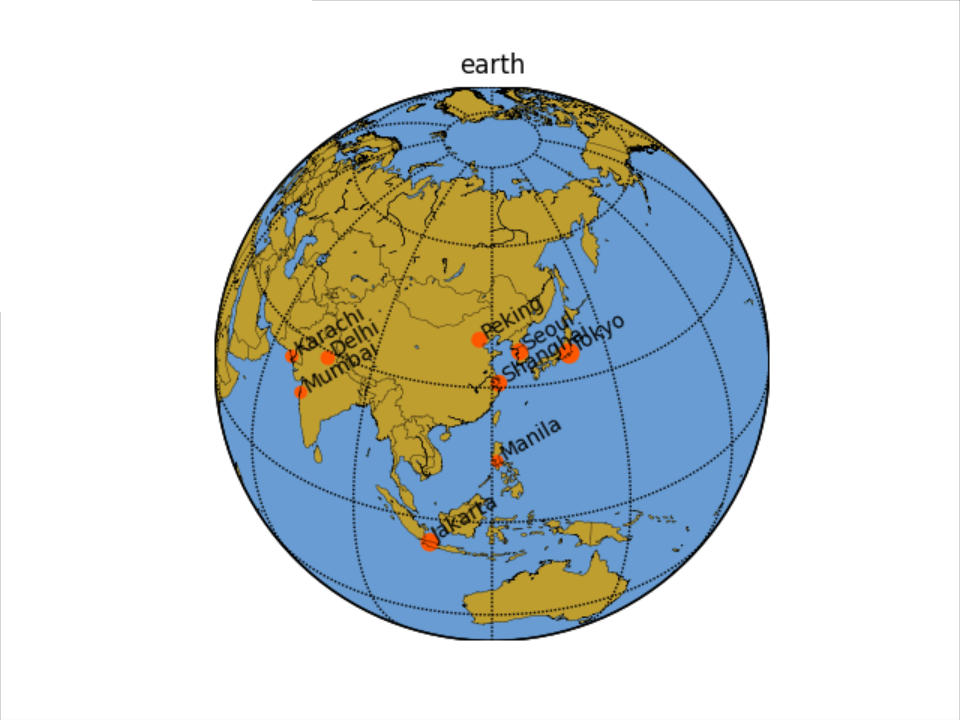### 画投影地球

 `12345678910111213` ``````def draw_earth1(): import matplotlib.pyplot as plt from mpl_toolkits.basemap import Basemap plt.figure(figsize=(8, 8)) # 正射投影，投影原点设在了上海周边 m = Basemap(projection='ortho', resolution=None, lat_0=30, lon_0=120) # 图像原始分辨率是5400*2700，设置scale = 0.5以后分辨率为2700*1350,如此作图 # 迅速不少也不那么占用内存了 m.bluemarble(scale=0.5) plt.show() if __name__ == '__main__': draw_earth1() ``````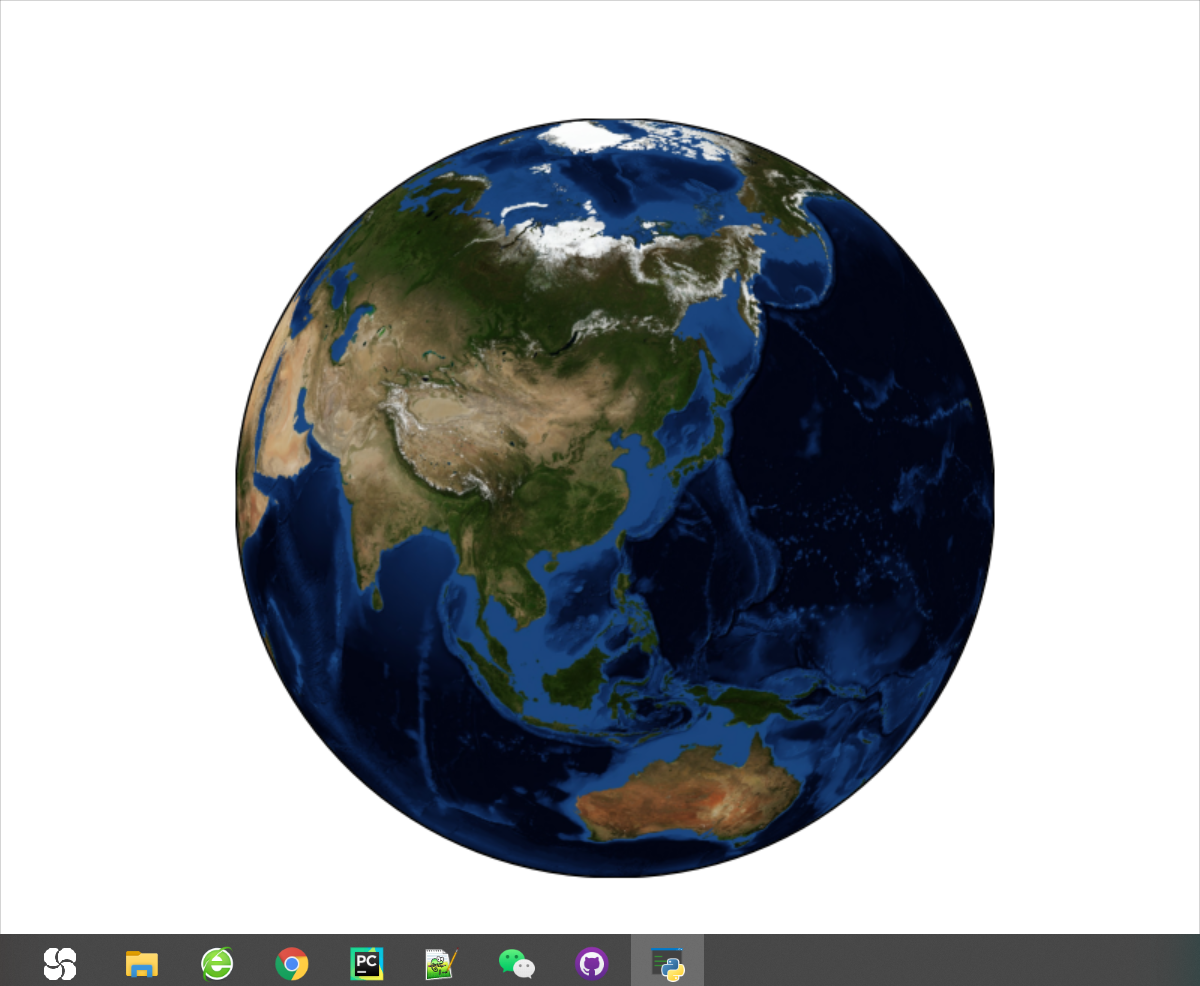### 总结